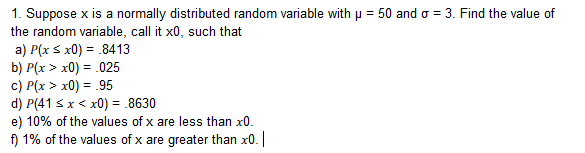# 1. Suppose x is a normally distributed random variable withthe random variable, call it x0, such thata) P(x x0)8413b) P(x > x0) = 025c) P(x > x0) = .95d) P(41 Sx < x0) 8630e) 10% of the values of x are less than x0.f) 1% of the values of x are greater than x50 and ơ-3. Find the value of0

Question
774 views

a.b.chelp_outlineImage Transcriptionclose1. Suppose x is a normally distributed random variable with the random variable, call it x0, such that a) P(x x0)8413 b) P(x > x0) = 025 c) P(x > x0) = .95 d) P(41 Sx < x0) 8630 e) 10% of the values of x are less than x0. f) 1% of the values of x are greater than x 50 and ơ-3. Find the value of 0 fullscreen
check_circle

Step 1

Standard normal distribution:

The standard normal distribution is a special case of normal distribution. The standard normal distribution will have mean 0 and standard deviation 1. If a random variable y follows normal distribution with mean (m) and standard deviation (s), then the standard normal variable z will be as given below:

Step 2

a.

Find the value of x0 for which P(x x0) = 0.8413:

The random variable x follows normal distribution with mean 50 and standard deviation 3.

That is, m = 50 and s = 3.

The value of x0 for which P(x x0) = 0.8413 is obtained as 53 from the calculation given below:

Step 3

b.

Find the value of x0 for which P(x > x0) = 0.025:

The value of x0 for which P(x > x0) = 0.025 is obtained as 58.88 from the calculation g...

### Want to see the full answer?

See Solution

#### Want to see this answer and more?

Solutions are written by subject experts who are available 24/7. Questions are typically answered within 1 hour.*

See Solution
*Response times may vary by subject and question.
Tagged in

### Statistics